CIVE 530 - OPEN-CHANNEL HYDRAULICS LECTURE 8B: GRADUALLY VARIED FLOW II

 8.3  CHARACTERISTICS OF FLOW PROFILES

• The gradually varied flow equation for hydraulically wide channels is:

 Sy/Sc = [(So/Sc) - F2] / (1 - F2)

• In the LHS of the gradually varied flow equation, Sc is always positive, because friction cannot be zero or negative.

• Therefore, the sign of the LHS will be that of Sy.

• The sign of the LHS can be either of three (3) possibilities:

1. Positive: RETARDED FLOW (BACKWATER).

2. Zero: UNIFORM FLOW (NORMAL).

3. Negative: ACCELERATED FLOW (DRAWDOWN).

• In the RHS, there are three (3) possibilities for the numerator:

1. (So/Sc) > F2 -----> SUBNORMAL

2. (So/Sc) = F2 -----> NORMAL

3. (So/Sc) < F2 -----> SUPERNORMAL

• In the RHS, there are three (3) possibilities for the denominator:

1. 1 > F2 -----> SUBCRITICAL

2. 1 = F2 -----> CRITICAL (the equation is undefined)

3. 1 < F2 -----> SUPERCRITICAL

• There are three types of families of profiles:

1. Type I: Positive.

Both numerator and denominator are positive -----> subnormal/subcritical flow -----> RETARDED FLOW.

2. Type II: Negative.

A. Numerator is positive and denominator is negative -----> subnormal/supercritical flow -----> ACCELERATED FLOW.

B. Numerator is negative and denominator is positive -----> supernormal/subcritical flow -----> ACCELERATED FLOW.

3. Type III: Positive.

Both numerator and denominator are negative -----> supernormal/supercritical flow -----> RETARDED FLOW.

• In a Type I family (subnormal/subcritical), the rule is:

 1 > F2 < (So/Sc)

which is the same as:

 (So/Sc) > < 1

(So/Sc can be greater, equal to, or less than 1)

• In the Type I family, there are three profiles:

1. M1 -----> So < Sc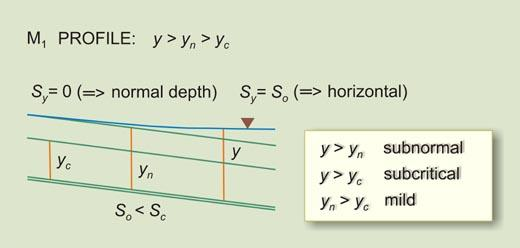2. C1 -----> So = Sc3. S1 -----> So > Sc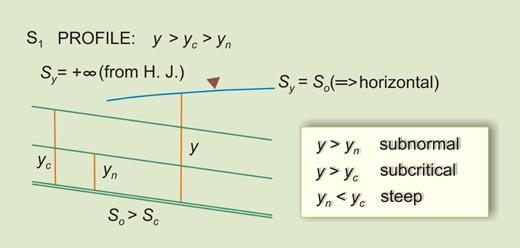• In a Type I family, there are not horizontal or adverse profiles because:

 (So/Sc) > F2 > 0

Therefore:

 So > 0

• In a Type IIA family (subnormal/supercritical), the rule is:

 1 < F2 < (So/Sc)

which is the same as:

 (So/Sc) > 1

(So/Sc can only be greater than 1)

• In the Type IIA family, there is one profile:

1. S2 -----> So > Sc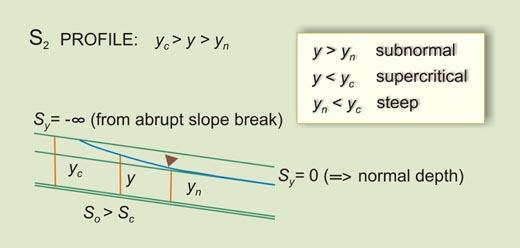• In a Type IIA family, there are not horizontal or adverse profiles because:

 (So/Sc) > F2 > 0

Therefore:

 So > 0

• In a Type IIB family (supernormal/subcritical), the rule is:

 1 > F2 > (So/Sc)

which is the same as:

 (So/Sc) < 1

(So/Sc can only be less than 1)

• In the Type IIB family, there are three profiles:

1. M2 -----> 0 < So < Sc2. H2 -----> 0 = So < Sc3. A2 -----> So < 0 < Sc• In a Type III family (supernormal/supercritical), the rule is:

 1 < F2 > (So/Sc)

which is the same as:

 (So/Sc) >< 1

(So/Sc can be greater, equal to, or less than 1)

• In the Type III family, there are five profiles:

1. S3 -----> So > Sc2. C3 -----> So = Sc3. M3 -----> 0 < So < Sc4. H3 -----> 0 = So < Sc5. A3 -----> So < 0 < ScTable 1. Summary of water surface profiles Family Character Rule So/Sc > 1 So/Sc = 1 So/Sc < 1 So = 0 So < 0 I Retarded (Backwater) 1 > F2 < (So/Sc) S1 C1 M1 - - IIA Accelerated (Drawdown) 1 < F2 < (So/Sc) S2 - - - - IIB Accelerated (Drawdown) 1 > F2 > (So/Sc) - - M2 H2 A2 III Retarded (Backwater) 1 < F2 > (So/Sc) S3 C3 M3 H3 A3

 8.4  LIMITS TO THE WATER SURFACE PROFILES

• The flow depth gradient is:

 dy/dx = (So - Sc F2) / (1 - F2)

 Sy = (So - Sc F2) / (1 - F2)

 Sy (1 - F2) = So - Sc F2

 Sy - Sy F2 = So - Sc F2

 F2 = (So - Sy) / (Sc - Sy)

• For uniform flow: Sy = 0. The previous equation leads to:

 So = Sc F2

• For gradually varied flow, the equation remains:

 F2 = (So - Sy) / (Sc - Sy)

• There are three cases:

• I.   F2 > 0

• II.   F2 = 0

• III.   F2 < 0

• Case I (F2 > 0) occurs when:

• So > Sy and Sc > Sy

The following inequality is satisfied: So > Sy < Sc

• So < Sy and Sc < Sy

The following inequality is satisfied: So < Sy > Sc

We conclude that Sy has to be either less than both So and Sc, or greater than both.

• Case II (F2 = 0) leads to So = Sy

 So = (z1 - z2)/L

 Sy = (y2 - y1)/L

 (z1 - z2)/L = (y2 - y1)/L

 z1 + y1 = y2 + z2

• This depicts a reservoir.A reservoir.

• Case III (F2 < 0) is impossible:

• So > Sy and Sy > Sc

The following inequality is NOT satisfied: So > Sy > Sc

• So < Sy and Sc > Sy

The following inequality is NOT satisfied: So < Sy < Sc

We conclude that Sy cannot be less than one and greater than the other slope (So and/or Sc).

Sy has to be either less than both So and Sc, or greater than both (see Case I).

• Case I (F2 > 0) can have three subcases:

• F2 < 1 -----> So - Sy < Sc - Sy

Leads to: So < Sc    subcritical flow

• F2 = 1 -----> So - Sy = Sc - Sy

Leads to: So = Sc    critical flow

• F2 > 1 -----> So - Sy > Sc - Sy

Leads to: So > Sc    supercritical flow

 Table 2. Behavior of depth gradient Sy. Channel slope RETARDED (BACKWATER), Sy is positive, decreasing in the direction of computation Type Name So < Sc Sy will decrease U/S from So (Horizontal) to 0 (Asymptotic to flow) I M1 So < Sc Sy will decrease U/S from +∞ (Vertical) to Sc (More than horizontal) III M3 So < Sc Sy will decrease U/S from +∞ (Vertical) to Sc (More than horizontal) III H3 So < Sc Sy will decrease U/S from +∞ (Vertical) to Sc (More than horizontal) III A3 So = Sc Sy will be constant and equal to So I C1 So = Sc Sy will be constant and equal to So III C3 So > Sc Sy will decrease D/S from +∞ (Vertical) to So (Horizontal) I S1 So > Sc Sy will decrease D/S from Sc (Less than horizontal) to 0 (Asymptotic to flow) III S3 Channel slope ACCELERATED (DRAWDOWN), Sy is NEGATIVE, increasing in the direction of computation Type Name So > Sc Sy will increase D/S from -∞ (Vertical) to 0 (Asymptotic to flow) IIA S2 So < Sc Sy will increase U/S from -∞ (Vertical) to 0 (Asymptotic to flow) IIB M2 So < Sc Sy will increase U/S from -∞ (Vertical) to So = 0 (Asymptotic to flow) IIB H2 So < Sc Sy will increase U/S from -∞ (Vertical) to So < 0 (Asymptotic to flow) IIB A2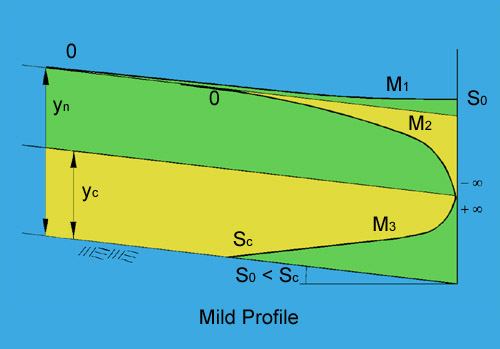Mild water-surface profiles.

 Table 3. (a) Occurrence of Water Surface Profiles: Mild channels M1 Flow in a mild channel upstream of a reservoir M2 Flow in a mild channel, upstream of an abrupt change in grade (or a steep channel carrying supercritical flow) M3 Flow in a mild channel, downstream of a steep channel carrying supercritical flow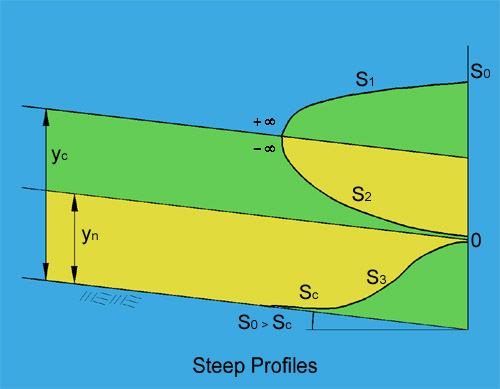Steep water-surface profiles.

 Table 3. (b) Occurrence of Water Surface Profiles: Steep channels S1 Flow in a steep channel upstream of a reservoir S2 Flow in a steep channel, downstream of a mild channel carrying subcritical flow S3 Flow in a steep channel, downstream of a steep channel carrying supercritical flow

 8.5  METHODOLOGIES TO CALCULATE WATER SURFACE PROFILES

There are two methods to calculate water-surface profiles:

1. the direct step method,

2. the standard step method.
These are compared in the following table.

 Table 4. Comparison between direct step and standard step methods. No. Characteristic Direct step method Standard step method 1 Ease of computation Easy (hours) Difficult (months) 2 Type of cross section prismatic any (prismatic or nonprismatic) 3 Independent variable flow depth length of channel 4 Dependent variable length of channel flow depth 5 Calculation advances -> directly by iteration (trial and error) 6 Accuracy increases with -> a smaller flow depth increment lesser cross-sectional variability 7 Type of cross-section input one typical cross section (prismatic) many cross sections (nonprismatic) 8 Data needs minimal extensive 9 Tools spread sheet (or programming) HEC-RAS 10 Reliability Answer always possible Answer sometimes not possible (depends on the type of cross-sectional data)

The number or required cross sections depends on:

• Channel slope: steeper channels may require more cross sections.

• Cross-sectional variability: the cross-sections need to reflect the longitudinal variability.

• Cost and project considerations.

• Modeling reality: modeling a three-dimensional flow phenomena as a one-dimensional (i.e, longitudinal description only) analog.

 060320